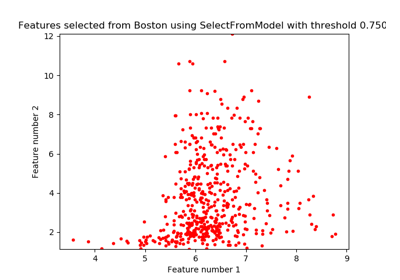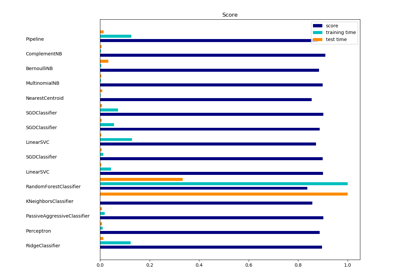# sklearn.feature_selection.SelectFromModel¶

class sklearn.feature_selection.SelectFromModel(estimator, threshold=None, prefit=False, norm_order=1, max_features=None)[source]

Meta-transformer for selecting features based on importance weights.

New in version 0.17.

Parameters
estimatorobject

The base estimator from which the transformer is built. This can be both a fitted (if prefit is set to True) or a non-fitted estimator. The estimator must have either a feature_importances_ or coef_ attribute after fitting.

thresholdstring, float, optional default None

The threshold value to use for feature selection. Features whose importance is greater or equal are kept while the others are discarded. If “median” (resp. “mean”), then the threshold value is the median (resp. the mean) of the feature importances. A scaling factor (e.g., “1.25*mean”) may also be used. If None and if the estimator has a parameter penalty set to l1, either explicitly or implicitly (e.g, Lasso), the threshold used is 1e-5. Otherwise, “mean” is used by default.

prefitbool, default False

Whether a prefit model is expected to be passed into the constructor directly or not. If True, transform must be called directly and SelectFromModel cannot be used with cross_val_score, GridSearchCV and similar utilities that clone the estimator. Otherwise train the model using fit and then transform to do feature selection.

norm_ordernon-zero int, inf, -inf, default 1

Order of the norm used to filter the vectors of coefficients below threshold in the case where the coef_ attribute of the estimator is of dimension 2.

max_featuresint or None, optional

The maximum number of features selected scoring above threshold. To disable threshold and only select based on max_features, set threshold=-np.inf.

New in version 0.20.

Attributes
estimator_an estimator

The base estimator from which the transformer is built. This is stored only when a non-fitted estimator is passed to the SelectFromModel, i.e when prefit is False.

threshold_float

The threshold value used for feature selection.

Notes

Allows NaN/Inf in the input if the underlying estimator does as well.

Examples

>>> from sklearn.feature_selection import SelectFromModel
>>> from sklearn.linear_model import LogisticRegression
>>> X = [[ 0.87, -1.34,  0.31 ],
...      [-2.79, -0.02, -0.85 ],
...      [-1.34, -0.48, -2.55 ],
...      [ 1.92,  1.48,  0.65 ]]
>>> y = [0, 1, 0, 1]
>>> selector = SelectFromModel(estimator=LogisticRegression()).fit(X, y)
>>> selector.estimator_.coef_
array([[-0.3252302 ,  0.83462377,  0.49750423]])
>>> selector.threshold_
0.55245...
>>> selector.get_support()
array([False,  True, False])
>>> selector.transform(X)
array([[-1.34],
[-0.02],
[-0.48],
[ 1.48]])


Methods

 fit(self, X[, y]) Fit the SelectFromModel meta-transformer. fit_transform(self, X[, y]) Fit to data, then transform it. get_params(self[, deep]) Get parameters for this estimator. get_support(self[, indices]) Get a mask, or integer index, of the features selected inverse_transform(self, X) Reverse the transformation operation partial_fit(self, X[, y]) Fit the SelectFromModel meta-transformer only once. set_params(self, \*\*params) Set the parameters of this estimator. transform(self, X) Reduce X to the selected features.
__init__(self, estimator, threshold=None, prefit=False, norm_order=1, max_features=None)[source]

Initialize self. See help(type(self)) for accurate signature.

fit(self, X, y=None, **fit_params)[source]

Fit the SelectFromModel meta-transformer.

Parameters
Xarray-like of shape (n_samples, n_features)

The training input samples.

yarray-like, shape (n_samples,)

The target values (integers that correspond to classes in classification, real numbers in regression).

**fit_paramsOther estimator specific parameters
Returns
selfobject
fit_transform(self, X, y=None, **fit_params)[source]

Fit to data, then transform it.

Fits transformer to X and y with optional parameters fit_params and returns a transformed version of X.

Parameters
Xnumpy array of shape [n_samples, n_features]

Training set.

ynumpy array of shape [n_samples]

Target values.

**fit_paramsdict

Returns
X_newnumpy array of shape [n_samples, n_features_new]

Transformed array.

get_params(self, deep=True)[source]

Get parameters for this estimator.

Parameters
deepbool, default=True

If True, will return the parameters for this estimator and contained subobjects that are estimators.

Returns
paramsmapping of string to any

Parameter names mapped to their values.

get_support(self, indices=False)[source]

Get a mask, or integer index, of the features selected

Parameters
indicesboolean (default False)

If True, the return value will be an array of integers, rather than a boolean mask.

Returns
supportarray

An index that selects the retained features from a feature vector. If indices is False, this is a boolean array of shape [# input features], in which an element is True iff its corresponding feature is selected for retention. If indices is True, this is an integer array of shape [# output features] whose values are indices into the input feature vector.

inverse_transform(self, X)[source]

Reverse the transformation operation

Parameters
Xarray of shape [n_samples, n_selected_features]

The input samples.

Returns
X_rarray of shape [n_samples, n_original_features]

X with columns of zeros inserted where features would have been removed by transform.

partial_fit(self, X, y=None, **fit_params)[source]

Fit the SelectFromModel meta-transformer only once.

Parameters
Xarray-like of shape (n_samples, n_features)

The training input samples.

yarray-like, shape (n_samples,)

The target values (integers that correspond to classes in classification, real numbers in regression).

**fit_paramsOther estimator specific parameters
Returns
selfobject
set_params(self, **params)[source]

Set the parameters of this estimator.

The method works on simple estimators as well as on nested objects (such as pipelines). The latter have parameters of the form <component>__<parameter> so that it’s possible to update each component of a nested object.

Parameters
**paramsdict

Estimator parameters.

Returns
selfobject

Estimator instance.

transform(self, X)[source]

Reduce X to the selected features.

Parameters
Xarray of shape [n_samples, n_features]

The input samples.

Returns
X_rarray of shape [n_samples, n_selected_features]

The input samples with only the selected features.

## Examples using sklearn.feature_selection.SelectFromModel¶